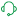# New to QlikView

Discussion board where members can get started with QlikView.

Announcements
Modernize Your QlikView Deployment webinar, Nov. 3rd. REGISTER
cancel
Showing results for
Did you mean:
HighlightedContributor III

## Calculating rate of sale

I need to calculate of  sale, number of units sold per week.if i select last year, then it shoould be retail quanity /52(number of weeks in a year). if i select current year from period filter, suppose current month is jan then calculation be like retail quantity/4(number of weeks in jan month) if current month is aug then calculation should be retail quanity/36(number weeks till august) and if the current month is oct then calculation be like retail quantity/40(number of weeks till oct). Attaching you the qvw file for your refrence.

I'm unsing the below code,with this i'm not getting the desried output. This problem is on the sales tab and ros text object in the dashboard

='Current Year:'& Num((Sum({<week = {">=\$(vMinDate)<=\$(vMaxdate)"}>} RTL_QTY)/Count(DISTINCT( week))/1000000),'##.00')
& ' Last Year:'&num((((Sum({<week = {">=\$(vRollingStart)<=\$(vRollingEnd)"},Period=>} RTL_QTY)/Count(DISTINCT( week))
-
Sum({<week = {">=\$(vRollingStart)<=\$(vRollingEnd)"},Period= {'LYTD'}> }RTL_QTY)/Count(DISTINCT( week)),'##.00')

4 Replies
HighlightedMVP

Something like this?

='Current Year:'& If(GetFieldSelections(Year)<>Max(TOTAL Year), Num((Sum({<week = {">=\$(vMinDate)<=\$(vMaxdate)"}>} RTL_QTY)/Count(DISTINCT( week))/1000000),'##.00'), Num((Sum({<week = {"<=\$(=Max(week))"}>} RTL_QTY)/Count(DISTINCT( week))/1000000),'##.00'))
& ' Last Year:'& If(GetFieldSelections(Year)<>Max(TOTAL Year), num((((Sum({<week = {">=\$(vRollingStart)<=\$(vRollingEnd)"},Period=>} RTL_QTY)/Count(DISTINCT( week))-
Sum({<week = {">=\$(vRollingStart)<=\$(vRollingEnd)"},Period= {'LYTD'}> }RTL_QTY)/Count(DISTINCT( week)),'##.00'), Num((Sum({<week = {"<=\$(=Max(week))"}>} RTL_QTY)/Count(DISTINCT( week))/1000000),'##.00'))

Before develop something, think If placed (The Right information | To the right people | At the Right time | In the Right place | With the Right context)
HighlightedContributor III

it's throwing a error in expression ')'expected with the below code

='Current Year:'& If(GetFieldSelections(Year)<>Max(TOTAL Year), Num((Sum({<week = {">=\$(vMinDate)<=\$(vMaxdate)"}>} RTL_QTY)/Count(DISTINCT( week))/1000000),'##.00'), Num((Sum({<week = {"<=\$(=Max(week))"}>} RTL_QTY)/Count(DISTINCT( week))/1000000),'##.00'))
& ' Last Year:'& If(GetFieldSelections(Year)<>Max(TOTAL Year), num((((Sum({<week = {">=\$(vRollingStart)<=\$(vRollingEnd)"},Period=>} RTL_QTY)/Count(DISTINCT( week))-
Sum({<week = {">=\$(vRollingStart)<=\$(vRollingEnd)"},Period= {'LYTD'}> }RTL_QTY)/Count(DISTINCT( week)),'##.00'), Num((Sum({<week = {"<=\$(=Max(week))"}>} RTL_QTY)/Count(DISTINCT( week))/1000000),'##.00'))

HighlightedContributor III

dataset and qvw file atttached

HighlightedDigital Support

Have a look at the following Design Blog post, believe that may be of some further help to you:

https://community.qlik.com/t5/Qlik-Design-Blog/A-Primer-on-Set-Analysis/ba-p/1468344

If you want to do some further looking around, use the following URL for that:

https://community.qlik.com/t5/Qlik-Design-Blog/bg-p/qlik-design-blog

This will also kick the post back up to where someone else may have another look at things too.

Regards,
Brett

To help users find verified answers, please do not forget to use the "Accept as Solution" button on any post(s) that helped you resolve your problem or question.
I now work a compressed schedule, Tuesday, Wednesday and Thursday, so those will be the days I will reply to any follow-up posts.